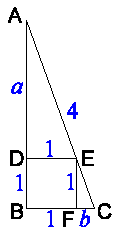The picture below shows the situation.Let a be the length of line segment AD, and let b be the length of line segment CF.

Because of the similarity of the triangles ADE and EFC, the following holds:

a : 1 = 1 : b

so

ab = 1.

According to the Pythagorean Theorem, the following holds:

(AB)2 + (BC)2 = (AC)2

so

(a + 1)2 + (1 + b)2 = 42

which can be rewritten to

a2 + 2 + b2 + 2(a + b) = 16.

Now we use the fact that ab=1, so 2=2ab, and we get:

a2 + 2ab + b2 + 2(a + b) = 16

which can be rewritten to

(a + b)2 + 2×(a + b) - 16 = 0.

Because we know that a+b is greater than 0, using the quadratic formula we find that

a + b = sqrt(17) - 1.

Because of the similarity of the triangles ADE and EFC, the following holds:

a : 1 = 1 : b

so

b = 1 / a.

Now we know that

a + 1/a = sqrt(17) - 1

so

a2 + ( 1 - sqrt(17) )×a + 1 = 0.

We know that a is greater than 0, and using the quadratic formula we find the following two values for a:

1/2×( sqrt(17) - 1 + sqrt( ( 1 - sqrt(17) )2 - 4 ) ) ≈ 2.76
1/2×( sqrt(17) - 1 - sqrt( ( 1 - sqrt(17) )2 - 4 ) ) ≈ 0.36

The ladder touches the wall 1 meter higher, which is at about 3.76 or 1.36 meters. In the figure, we can see that only the answer 3.76 can be correct.Back to the puzzle